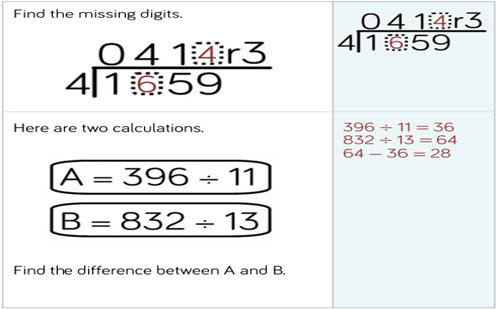This guide provides a sequence of small steps starting with a recap of different addition, subtraction, multiplication and division strategies before moving onto factors, multiples, long division, primes to 100, and more.

Year level(s) Year 4, Year 5, Year 6
Audience Teacher
Purpose Teaching resource, Teaching strategies
Teaching strategies and pedagogical approaches Questioning, Concrete Representational Abstract model, Explicit teaching
Keywords area model, factors, multiples, common factors, common multiples, prime numbers, explicit teaching

## Curriculum alignment

Curriculum connections Numeracy
Strand and focus Number, Build understanding
Topics Addition and subtraction, Multiplication and division, Multiples, factors and powers, Operating with number, Place value
AC: Mathematics (V9.0) content descriptions
AC9M4N06
Develop efficient strategies and use appropriate digital tools for solving problems involving addition and subtraction, and multiplication and division where there is no remainder

AC9M5N06
Solve problems involving multiplication of larger numbers by one- or two-digit numbers, choosing efficient calculation strategies and using digital tools where appropriate; check the reasonableness of answers

AC9M5N07
Solve problems involving division, choosing efficient strategies and using digital tools where appropriate; interpret any remainder according to the context and express results as a whole number, decimal or fraction

AC9M6N02
Identify and describe the properties of prime, composite and square numbers and use these properties to solve problems and simplify calculations

AC9M6N09
Use mathematical modelling to solve practical problems, involving rational numbers and percentages, including in financial contexts; formulate the problems, choosing operations and efficient calculation strategies, and using digital tools where appropriate; interpret and communicate solutions in terms of the situation, justifying the choices made

AC9M6N01
Recognise situations, including financial contexts, that use integers; locate and represent integers on a number line and as coordinates on the Cartesian plane

Numeracy progression Additive strategies (P8, P9)
Multiplicative strategies (P7, P8, P9)
Number and place value (P7, P9)
Interpreting fractions (P8)
Understanding money (P8)
Positioning and locating (P5)